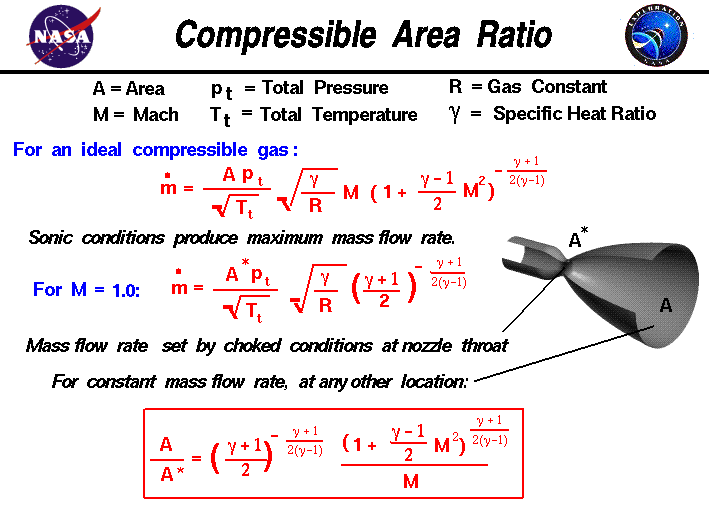+ Text Only Site
+ Non-Flash Version
+ Contact GlennThe conservation of mass is a fundamental concept of physics. Within some problem domain, the amount of mass remains constant; mass is neither created or destroyed. The mass of any object is simply the volume that the object occupies times the density of the object. For a fluid (a liquid or a gas) the density, volume, and shape of the object can all change within the domain with time and mass can move through the domain.

The conservation of mass (continuity) tells us that the mass flow rate mdot through a tube is a constant. If we include compressibility effects for high speed flow through a duct, we find that the mass flow rate depends on the flow area A, total pressure pt and temperature Tt of the flow, the Mach number M, the ratio of specific heats of the gas gam, and the gas constant R:

mdot = (A * pt/sqrt[Tt]) * sqrt(gam/R) * M * [1 + M^2 * (gam-1)/2]^-[(gam+1)/(gam-1)/2]

The compressibility effects on mass flow rate have some unexpected results. We can increase the mass flow through a tube by increasing the area, increasing the total pressure, or decreasing the total temperature. But the effect of increasing velocity (Mach number) is a little harder to figure out. If we were to fix the area, total pressure and temperature, and graph the variation of mass flow rate with Mach number, we would find that a limiting maximum value occurs at Mach number equal to one. Using calculus, we can mathematically determine the same result: there is a maximum airflow limit that occurs when the Mach number is equal to one. The limiting of the mass flow rate is called choking of the flow. The value of the mass flow rate at choked conditions is given by:

mdot = (A* * pt/sqrt[Tt]) * sqrt(gam/R) * [(gam+1)/2]^-[(gam+1)/(gam-1)/2]

Mach number equal to one is called a sonic condition because the velocity is equal to the speed of sound and we denote the area for the sonic condition by "A*".

If we have a tube with changing area, like the nozzle shown on the slide, the maximum mass flow rate through the system occurs when the flow is choked at the smallest area. This location is called the throat of the nozzle. The conservation of mass specifies that the mass flow rate through a nozzle is a constant. If no heat is added, and there are no pressure losses in the nozzle, the total pressure and temperature are also constant. By substituting the sonic conditions into the mass flow rate equation, and doing some algebra, we can relate the Mach number M at any location in the nozzle to the ratio between the area A at that location and the area of the throat A*. The resulting equation is shown in the box at the bottom of the slide.

A / A* = {[(gam+1)/2]^-[(gam+1)/(gam-1)/2]} / M * [1 + M^2 * (gam-1)/2]^[(gam+1)/(gam-1)/2]

Here is a JavaScript program that solves the area ratio equation.

# Isentropic Flow Calculator

Input
Output
Mach
Mach Angle
P-M Ang
p/pt
T/Tt
rho/rhot
q/p
A/A*
Wcor/A

By default, the program Input Variable is the Mach number of the flow. Since the area ratio depends only on the Mach number and ratio of specific heats, the program can calculate the value of the area ratio and display the results on the right side of the output variables. You can also have the program solve for the Mach number that produces a desired value of area ratio. Using the choice button labeled Input Variable, select "Area Ratio - A/A*". Directly below the selection, you then type in a value for A/A*. When you hit the Enter key on your keyboard, the output values change. The area ratio is double valued; for the same area ratio, there is a subsonic and a supersonic solution. The choice button at the right top selects the solution that is presented.

If you are an experienced user of this calculator, you can use a sleek version of the program which loads faster on your computer and does not include these instructions. You can also download your own copy of the program to run off-line by clicking on this button:

Considering a rocket nozzle, we can set the mass flow rate by setting the area of the throat. And we can set the exit Mach number by setting the area ratio of the exit to the throat. Using the isentropic relations, we can determine the pressure and temperature at the exit of the nozzle. And from the Mach number and temperature we can determine the exit velocity. If we consider the rocket thrust equation, we have now determined all the values necessary to determine the thrust of the rocket. You can explore the operation of a nozzle with our interactive thrust simulator and design your own rockets!

Guided Tours
Activities:

Related Sites:
Rocket Index
Rocket Home
Beginner's Guide Home+ Inspector General Hotline + Equal Employment Opportunity Data Posted Pursuant to the No Fear Act + Budgets, Strategic Plans and Accountability Reports + Freedom of Information Act + The President's Management Agenda + NASA Privacy Statement, Disclaimer, and Accessibility CertificationEditor: Tom Benson NASA Official: Tom Benson Last Updated: May 13 2021 + Contact Glenn